# AC Through Resistor And Inductor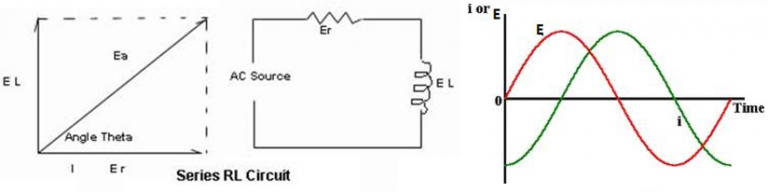Let a pure inductance and a pure resistance be connected in series to an alternating emf. Let Ebe the rms value of applied emf and I be the rms value of the current flowing in the circuit. Let VR and VL be the potential drop across R and L respectively.
The potential drop across inductor is: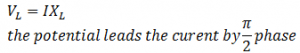The potential drop across resistance is: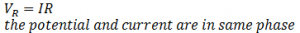Since the potential leads the current by π/2 phase in inductive circuit so the potential has been represented by OB at π/2 of OA
Since the potential and the current are in the same phase in case of resistive circuit so they have been represented by the same line OA.

From the fig the resultant OH is given by: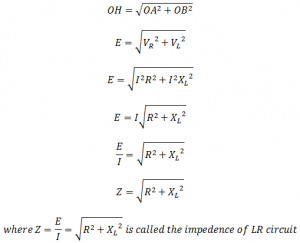This is the effective opposition offered by LR circuit for the flow of current.
Also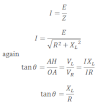This shows that the potential leads the current by π/2 in LR circuit.

Since the potential lags the current by π/2 phase in case of capacitor circuit so the potential has been represented by OA at π/2 of OB

Since the potential and the current are in the same phase in case of resistive circuit so they have been represented by the same line OB.